CiteScore 2.2
More on impact ›

# Frontiers in Built Environment## ORIGINAL RESEARCH article

Front. Built Environ., 09 June 2020 | https://doi.org/10.3389/fbuil.2020.00062

# Hysteretic–Viscous Hybrid Damper System for Long-Period Pulse-Type Earthquake Ground Motions of Large AmplitudeShoki Hashizume and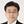Izuru Takewaki*
• Department of Architecture and Architectural Engineering, Graduate School of Engineering, Kyoto University, Kyoto, Japan

This paper aims to develop a hysteretic–viscous hybrid (HVH) damper system for long-period pulse-type earthquake ground motions of large amplitude. Long-period pulse-type earthquake ground motions of large amplitude have been recorded recently (Northridge, 1994; Kumamoto, 2016). It is well-known that these ground motions could cause severe damage to high-rise and base-isolated buildings with long natural period. To mitigate the damage caused by such ground motion, a new viscous–hysteretic hybrid damper system is proposed here, which consists of a viscous damper with large stroke and a hysteretic damper including a gap mechanism. A double impulse is employed as a representative of long-period pulse-type earthquake ground motions of large amplitude and a closed-form maximum response to this double impulse is derived for an elastic–plastic SDOF system including the proposed HVH system. To reveal the effectiveness of the proposed HVH system, time-history response analyses are performed for an amplitude modulated double impulse and a recorded ground motion at Kumamoto (2016). The performance comparison with the previous dual hysteretic damper (DHD) system consisting of small-amplitude and large-amplitude hysteretic dampers in parallel is also conducted to investigate the effectiveness of the proposed HVH system.

## Introduction

In the field of structural engineering of buildings and infrastructures, the resilience of structures is attracting many researchers and being treated as one of the targets of structural design (Bruneau et al., 2003; Cimellaro et al., 2010; Takewaki et al., 2011; Noroozinejad et al., 2019). The resilience consists of two phases, i.e., the resistance to disturbances and the recovery from damages. While the resistance can mostly be dealt with properly by the structural engineering technology, the recovery is related to various multidisciplinary fields including non-structural engineering fields.

Up to now, various innovative methodologies for upgrading the level of resilience have been exploited. The structural control is a well-accepted reliable strategy in terms of cost and implementability (Aiken et al., 1993; Hanson, 1993; Nakashima et al., 1996; Soong and Dargush, 1997; Hanson and Soong, 2001; Takewaki, 2009; Lagaros et al., 2013). The control of earthquake response by passive dampers certainly enables the upgrade of earthquake resilience levels and the continuous use of buildings (Taniguchi et al., 2016a). In the 2016 Kumamoto earthquake (Japan), severe shakings were observed repeatedly within 2 days and JMA (Japan Metheorological Agency) seismic intensity 7 (the highest level in the JMA scale; approximately X–XII in Mercalli scale) was recorded. As a result, unprecedented large-amplitude ground motions, called long-period pulse-type ground motions, were recorded. Even for such large-amplitude ground motions, the suppression of plastic deformations is strongly recommended in view of the resistance and recovery as the measure of earthquake resilience (Kojima and Takewaki, 2016; Ogawa et al., 2017).

It is well-recognized that the sophisticated and smart use of passive dampers is extremely important because their effectiveness strongly depends on the quantity and location. For responding properly to this requirement, various innovative methods have been proposed (see, for example, Xia and Hanson, 1992; Inoue and Kuwahara, 1998; Quagliarella et al., 1998; Uetani et al., 2003; Aydin et al., 2007; Takewaki, 2009; Aittokoski and Miettinen, 2010; Lavan and Levy, 2010; Adachi et al., 2013a,b; Lagaros et al., 2013; Fujita et al., 2014). As for the design of linear and non-linear viscous dampers, various useful, and effective methods have been proposed (Uetani et al., 2003; Attard, 2007; Aydin et al., 2007; Takewaki, 2009; Lavan and Levy, 2010; Adachi et al., 2013a,b; Noshi et al., 2013). To overcome the cost problem of viscous dampers (Murakami et al., 2013a), hysteretic dampers, such as buckling-restrained ones, have often been used in many buildings. At the same time, a problem is discussed recently resulting from their complex characteristics (Uetani et al., 2003; Murakami et al., 2013a,b). The non-linear characteristics of hysteretic dampers are similar to those of friction-damped types (Pall and Marsh, 1982; Austin and Pister, 1985; Filiatrault and Cherry, 1990; Cherry and Filiatraut, 1993; Ciampi et al., 1995). In addition, since hysteretic dampers exhibit residual deformation, complex hysteretic rules are required in the response evaluation.

As for hysteretic dampers, Inoue and Kuwahara (1998) treated a single-degree-of-freedom (SDOF) model and established a criterion on the optimal hysteretic damper quantity in terms of the equivalent viscous damping (Caughey, 1960; Jacobsen, 1960). Furthermore, Lavan and Levy (2010) developed an optimal design method by taking advantage of a newly derived optimality condition. Murakami et al. (2013a,b) proposed a general and stable sensitivity-based approach applicable to various kinds of dampers. Sivandi-Pour et al. (2014) investigated the equivalent modal damping ratios for non-classically damped hybrid steel concrete buildings.

Because hysteretic dampers possess abovementioned peculiar characteristics, most past researches on hysteretic dampers required numerical optimization algorithms including time-history response analysis for response evaluation and tremendous amount of computational effort was required to reveal special properties of the optimal damper location and quantity. On the other hand, Shiomi et al. (2016) proposed a novel design method for hysteretic dampers using an explicit expression of the maximum elastic–plastic response of an SDOF system with hysteretic dampers under the critical near-fault ground motion that is modeled by “the double impulse” (Kojima and Takewaki, 2015; Taniguchi et al., 2016b). Then, an explicit optimization was performed using this explicit expression. However, the performance comparison with other-type passive dampers has never been conducted under earthquake ground motions with broad amplitude.

In this paper, a hysteretic–viscous hybrid (HVH) damper system is proposed for long-period pulse-type earthquake ground motions of large amplitude, which consists of a viscous damper and a hysteretic damper with a gap mechanism (Tagawa and Hou, 2008; Asakawa et al., 2017). It is demonstrated that HVH is effective for large-amplitude input motions expressed by the double impulse.

## Double Impulse as Representative of Main Part of Near-Fault Ground Motion

Kojima and Takewaki (2015) demonstrated that the double impulse is a good substitute of the main part of a near-fault ground motion. They introduced the double impulse based on the motivation such that, while the normal input, such as a sinusoidal input or earthquake ground motions, requires the combination of a free-vibration component and a forced-vibration component for their elastic linear responses, the double impulse induces only a free-vibration component. This enables the avoidance to encounter the transcendental equation for finding the maximum response and the efficient use of the energy balance law for deriving the maximum response without time-history response analysis. In the introduction of the double impulse, the principal part of a near-fault ground motion is first modeled by a one-cycle sine wave üg sin(t) as shown in Equation (1) (see Figure 1A) and then transformed into a double impulse ügimp(t) expressed by Equation (2) (see Figure 1B).

In Equations (1) and (2), Ap, ωp, V, and t0 indicate the acceleration amplitude of the one-cycle sine wave, the circular frequency of the one-cycle sine wave, the velocity amplitude of the double impulse, and the time interval of the two impulses, respectively. Kojima and Takewaki (2015) employed the condition of the same maximum Fourier amplitude in this transformation.

FIGURE 1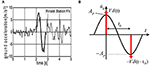Figure 1. Transformation of ground motion into double impulse. (A) Modeling of principal part of Rinaldi station FN motion (Northridge, 1994) into one-cycle sinusoidal wave; (B) Re-modeling into double impulse (Shiomi et al., 2018).

## Proposed HVH Damper

A new HVH damper system is proposed in this paper. In this system, a hysteretic damper with a gap mechanism in series and a viscous damper are used in parallel. The gap mechanism plays a role to give a trigger function to the hysteretic damper. As a result, this hysteretic damper with a gap mechanism possesses a function as a stopper.

### Mechanical Model

The model of a building structure including the proposed HVH system is shown in Figure 2. It is assumed that the building structure and the hysteretic damper have the elastic–perfectly plastic restoring-force characteristics. In this figure, KF, kL, c denote the frame stiffness, the stiffness ratio of the hysteretic damper to the frame, and the damping coefficient of the viscous damper.

FIGURE 2

### Mechanism of Response Reduction

The viscous damper is aimed at resisting for broad-amplitude range vibration and the hysteretic damper with a gap mechanism is expected to play as a stopper for large-amplitude range vibration.

## Closed-Form Critical Elastic–Plastic Response of Building Model With HVH

Kojima and Takewaki (2015) derived a closed-form expression of the maximum deformation of an elastic–perfectly plastic SDOF system under the critical double impulse by using an energy balance approach. This energy balance approach can be applied to more general models with broader class of restoring-force characteristics (see Shiomi et al., 2016, 2018). In this paper, a more general case is treated where a hysteretic damper with a gap mechanism and a viscous damper are used in parallel in an elastic–perfectly plastic SDOF system.

The model used in this paper is the SDOF model. Therefore, the effect of higher modes on the response of buildings is not considered. Since only the critical input of double impulse resonant to the elastic–plastic building with the HVH damper system is treated, the lowest-mode response governs most components of the total response of the building. This is because the long-period pulse-type earthquake ground motion possesses a clear predominant period and the treatment of the resonant input to the building is considered to be important in the investigation of the safety of the building.

The closed-form expressions are classified into several cases depending on the input level and structural parameters (see Table 1). In this case, the maximum displacement umax 1 after the first impulse, the velocity vc at the zero restoring force (frame plus hysteretic damper) after the first impulse, and the maximum displacement umax 2 after the second impulse can be derived.

TABLE 1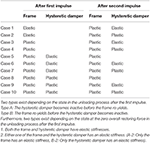Table 1. Classification of closed-form expressions on maximum deformation of the elastic–perfectly plastic SDOF system with HVH damper system under critical double impulse.

In the present model, an example of the restoring-force characteristic is presented in Figure 3 and the same energy balance approach can be applied by referring to the restoring-force and damping-force diagrams as shown in Figure 4, i.e., Cases 1–4 in Figure 4A, Case 5 in Figure 4B, Case 6 in Figure 4C, Case 7 in Figure 4D, Case 8 in Figure 4E, Case 9 in Figure 4F, and Case 10 in Figure 4G. When a viscous damper exists, an extended energy balance approach by Kojima et al. (2018) can be used for closed-form expressions.

FIGURE 3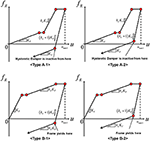Figure 3. Classification depending on the state in the unloading process after the first impulse and the state at the zero overall restoring force in the unloading process after the first impulse (Cases 5–10).

FIGURE 4A
FIGURE 4B
FIGURE 4C
FIGURE 4D
FIGURE 4E
FIGURE 4F
FIGURE 4G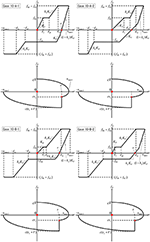Figure 4G. (A) Restoring-force and damping-force diagrams for various closed-form expressions (Cases 1–4) on maximum deformation of elastic–perfectly plastic SDOF system with HVH damper system under critical double impulse (: 1st impulse;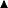: 2nd impulse). (B) Restoring-force and damping-force diagrams for various closed-form expressions (Case 5) on maximum deformation of elastic–perfectly plastic SDOF system with HVH damper system under critical double impulse (: 1st impulse;: 2nd impulse). (C) Restoring-force and damping-force diagrams for various closed-form expressions (Case 6) on maximum deformation of elastic–perfectly plastic SDOF system with HVH damper system under critical double impulse (: 1st impulse;: 2nd impulse). (D) Restoring-force and damping-force diagrams for various closed-form expressions (Case 7) on maximum deformation of elastic–perfectly plastic SDOF system with HVH damper system under critical double impulse (: 1st impulse;: 2nd impulse). (E) Restoring-force and damping-force diagrams for various closed-form expressions (Case 8) on maximum deformation of elastic–perfectly plastic SDOF system with HVH damper system under critical double impulse (: 1st impulse;: 2nd impulse). (F) Restoring-force and damping-force diagrams for various closed-form expressions (Case 9) on maximum deformation of elastic–perfectly plastic SDOF system with HVH damper system under critical double impulse (: 1st impulse;: 2nd impulse). (G) Restoring-force and damping-force diagrams for various closed-form expressions (Case 10) on maximum deformation of elastic–perfectly plastic SDOF system with HVH damper system under critical double impulse (: 1st impulse;: 2nd impulse).

The closed-form expressions of the maximum displacement umax 1 after the first impulse, the velocity vc at the zero restoring force (frame plus hysteretic damper) after the first impulse, and the maximum displacement umax 2 after the second impulse for Cases 1–4 are as follows.

[Case 1]

[Case 2]

[Case 3]

[Case 4]

The closed-form expressions for Case 5 [A-1]–Case 10 [B-1] are shown in Appendix (Supplemental file). Since Case 10 [B-2] is a case including a general restoring-force characteristic, a detailed derivation for Case 10-B-2 is shown below to explain the derivation process of the closed-form expressions.

[Case 10] [B-2]

Consider the case where the frame and the hysteretic damper with a gap mechanism yield after both the first impulse and the second impulse.

Evaluate the work done by the viscous damper by approximating the damping force–deformation relation as a quadratic function. The damping force–deformation relation after the first impulse can be approximated by a quadratic function with vertex (u, fD) = (umax 1, 0) and passing through the point (u, fD) = (0, cV). fD can then be obtained as follows.

The work done by the damping force after the first impulse can be obtained by integrating Equation (3) from u = 0 to u = umax 1.

By using Equation (4), the energy balance law after the first impulse (see Figure 5) leads to

From Equation (5), umax 1 can be evaluated by

Derive the velocity vc at the timing of the second impulse based on the assumption that the critical timing of the second impulse (the timing of the second impulse maximizing the maximum response umax 2 after the second impulse with respect to a variable impulse timing) is the timing when the overall story shear force becomes zero.

FIGURE 5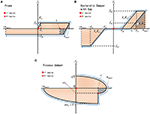Figure 5. Diagrams for Case 10-B-2. (A) Restoring force–deformation relation of frame; (B) Restoring force–deformation relation of hysteretic damper with gap mechanism; (C) Damping force–deformation relation of viscous damper.

Designate the deformation at the time when the overall story shear force becomes zero after the achievement of umax 1 as x. Then, Case 10 B-2 in Figure 4G for the restoring-force characteristic provides the following relation.

From Equation (7), the deformation x at the time when the overall story shear force becomes zero after the achievement of umax 1 can be expressed by

As in the previous case, the work done by the damping force is derived by using the quadratic function approximation. The damping force–deformation relation after achieving umax 1 is approximated by a quadratic function with vertex (u, fD) = (umax 1, 0) and passing through the point (u, fD) = (x, cvc). fD can be obtained as follows.

By integrating Equation (9) from u = x to u = umax 1, the work done by the damping force can be evaluated by

Equation (10) and the energy balance law lead to

From Equation (11), the velocity vc at the time when the overall shear force becomes zero can be expressed by

As in the above case, the damping force–deformation relation after the second impulse is approximated by a quadratic function with vertex (u, fD) = (−umax 2, 0) and passing through the point (u, fD) = (x, −c(vc + V)). fD can be obtained as follows.

By integrating Equation (13) from u = −umax 2 to u = x, the work done by the damping force can be evaluated by

Equation (14) and the energy balance law lead to

From Equation (15), umax 2 can be evaluated by

## Investigation on Accuracy of Closed-Form Expressions for Maximum Deformation of SDOF Building Model Including HVH System With Various Parameters

Consider an SDOF building model including the HVH system with various parameters. Figure 6 shows the comparison of the maximum deformation by the proposed closed-form expressions including an approximate damping force–deformation relation and by the time-history response analysis for the quantity kL = 1 of hysteretic dampers and the damping ratios h = 0, 0.05, 0.1, 0.2 of viscous dampers. Vy in the horizontal axis indicates the input velocity level of the double impulse such that the undamped model just reaches the yield level after the first impulse. In Figure 6, the maximum deformations after the first and second impulses are also plotted for reference. It should be remarked that, while the maximum deformation umax 1 after the first impulse becomes the maximum deformation umax in larger input velocity levels, the maximum deformation umax 2 after the second impulse becomes the maximum deformation umax in smaller input velocity levels. It can also be observed that the proposed closed-form expressions provide fairly accurate results for various damping levels and input levels.

FIGURE 6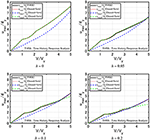Figure 6. Comparison of maximum deformation under critical double impulse between time-history response analysis and closed-form expression (kL = 1).

Figure 7 indicates the same comparison for the quantity kL = 2 of hysteretic dampers and the damping ratios h = 0, 0.05, 0.1, 0.2 of viscous dampers. It can also be seen that the proposed closed-form expressions possess fairly good accuracy. However, as the damping level becomes larger, a little difference appears.

FIGURE 7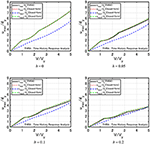Figure 7. Comparison of maximum deformation under critical double impulse between time-history response analysis and closed-form expression (kL = 2).

## Response Comparison of SDOF Building Model Including HVH System With Model Including DHD (Dual Hysteretic Damper) System Under Double Impulse

Figure 8A shows the elastic–perfectly plastic SDOF system with HVH damper system and one with DHD system that was proposed by Shiomi et al. (2018). In this figure, KF, k, α denote the frame stiffness, the stiffness ratio of DSA (hysteretic damper for small-amplitude control) to the frame, and the stiffness ratio of DLA (hysteretic damper for large-amplitude control) to DSA. It should be remarked that αk = kL in the model with HVH.

FIGURE 8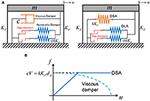Figure 8. Proposed model and comparison with previously developed model. (A) Elastic–perfectly plastic SDOF system with HVH damper system and one with DHD system; (B) Parameter adjustment between HVH and DHD.

In comparing the response reduction performances by the HVH system and the DHD system, it is necessary to adjust the quantities of both systems. In this paper, cV = kKFdsy is employed as shown in Figure 8B. From this relationship, it can be understood that, as the parameter V becomes larger, the quantity of hysteretic dampers in the HVH and DHD systems increases for a given damping coefficient c of viscous dampers in the HVH system.

Figure 9 shows the comparison of the maximum deformations under the critical double impulse between the elastic–perfectly plastic SDOF system with HVH and one with DHD (α = 0) for h = 0.05, 0.1, 0.2, 0.3. The quantitative changes of the response of the model with HVH from that of the model with DHD are indicated in percent at the two input levels V/Vy = 2, 4. It should be reminded that, since αk = kL, α = 0 indicates the SDOF models with only the viscous damper in HVH and the short-amplitude hysteretic damper in DHD. It can be seen that the viscous damper is effective for the input of smaller level (V/Vy < 3). In addition, the quantity of the short-amplitude hysteretic damper in DHD is specified by using the relation cV = kKFdsy explained above. The good response reduction performance of the DHD system in V/Vy > 3 is due to the fact that, as the parameter V becomes larger, the quantity of hysteretic dampers in the DHD systems increases for a given damping coefficient c of viscous dampers in the HVH system. On the other hand, Figure 10 presents the same comparison for α = 1 and Figure 11 illustrates the comparison for α = 3. It can be observed that the HVH has a good response reduction performance in the broad range of input levels compared with the DHD.

FIGURE 9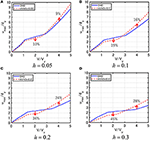Figure 9. Comparison of maximum deformation under critical double impulse between elastic–perfectly plastic SDOF system with HVH and one with DHD (α = 0) (A) h = 0.05, (B) h = 0.1, (C) h = 0.2, (D) h = 0.3.

FIGURE 10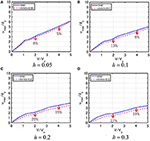Figure 10. Comparison of maximum deformation under critical double impulse between elastic–perfectly plastic SDOF system with HVH and one with DHD (α = 1) (A) h = 0.05, (B) h = 0.1, (C) h = 0.2, (D) h = 0.3.

FIGURE 11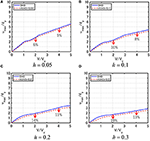Figure 11. Comparison of maximum deformation under critical double impulse between elastic–perfectly plastic SDOF system with HVH and one with DHD (α = 3) (A) h = 0.05, (B) h = 0.1, (C) h = 0.2, (D) h = 0.3.

## Response Comparison of SDOF Building Model Including the HVH System With Model Including DHD System Under Recorded Ground Motion

The effectiveness of the HVH system under a recorded ground motion of long-period pulse-type is shown in this section. Figure 12A and B show a ground acceleration and its velocity of JMA Nishiharamura–Komori(EW) wave during the Kumamoto earthquake in 2016, which is known as a long-period pulse-type ground motion of a very large velocity amplitude. The displacement, velocity, and acceleration response spectra are presented in Figures 12C–E. It can be found that this wave has a large velocity response around 0.7, 3.0(s).

FIGURE 12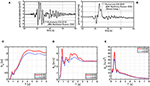Figure 12. Long-period pulse-type ground motion. (A) Ground acceleration of JMA Nishiharamura-Komori (EW) wave; (B) Ground velocity of JMA Nishiharamura–Komori(EW) wave; (C) Displacement response spectrum; (D) Velocity response spectrum; (E) Acceleration response spectrum.

Figure 13 indicates the comparison of the maximum deformation under the JMA Nishiharamura–Komori(EW) wave between the elastic–perfectly plastic SDOF system with HVH (structural damping ratio = 0) and one with DHD (structural damping ratio = 0) for the damping ratio h = 0.05, 0.1, 0.2, 0.3 of the viscous damper. V in the horizontal axis indicates the parameter used for cV = kKFdsy, which specifies the quantity of hysteretic dampers in DHD. Furthermore, the quantities of large-amplitude hysteretic dampers in DHD and hysteretic dampers in HVH are also given by this parameter V. The frame damped fundamental natural period = 2.7[s]. The deformation time histories are also plotted for reference (V/Vy = 1.3, V = 2.0[m/s], Vy = 1.57[m/s]). Furthermore, Figure 14 shows the same comparison for the structural damping ratio = 0.02. The frame damped fundamental natural period = 2.7005[s]. The deformation time histories are also plotted again for reference (V/Vy = 1.3, V = 2.0[m/s], Vy = 1.57[m/s]).

FIGURE 13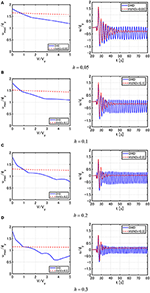Figure 13. Comparison of maximum deformation under JMA Nishiharamura–Komori(EW) wave between elastic–perfectly plastic SDOF system with HVH and one with DHD (structural damping ratio = 0) (A) h = 0.05, (B) h = 0.1, (C) h = 0.2, (D) h = 0.3.

FIGURE 14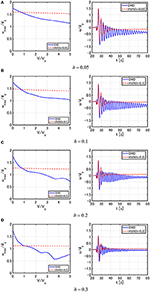Figure 14. Comparison of maximum deformation under JMA Nishiharamura–Komori(EW) wave between elastic–perfectly plastic SDOF system with HVH and one with DHD (structural damping ratio = 0.02) (A) h = 0.05, (B) h = 0.1, (C) h = 0.2, (D) h = 0.3.

It can be seen from Figures 13, 14 that the HVH system exhibits a stable performance compared to the DHD system. The good response reduction performance of the DHD system in the larger level of V/Vy is due to the fact that, as the parameter V becomes larger, the quantity of hysteretic dampers in the DHD systems increase for a given damping coefficient c of viscous dampers in the HVH system. It can also be observed that, while the SDOF system with DHD exhibits a fairly large residual deformation, the SDOF system with HVH does not induce large residual deformation.

The HVH damper system consists of viscous dampers (oil dampers) and hysteretic dampers with gap mechanism in parallel. Each damper has already been used in actual buildings in many countries. Therefore, it seems possible to use the HVH damper system in actual buildings.

## Conclusions

A new HVH damper system has been proposed for long-period pulse-type earthquake ground motions of large amplitude. The proposed system includes a viscous damper and a hysteretic damper with a gap mechanism. The following conclusions have been derived.

Although the structural control system is generally understood to be rather ineffective for impulsive earthquake ground motions, the viscous damper is aimed at resisting for broad-amplitude range vibration and the hysteretic damper with a gap mechanism is expected to play as a stopper for large-amplitude range vibration in the proposed damper system.

A closed-form maximum response to the critical double impulse with the impulse timing maximizing the response has been derived for an elastic–perfectly plastic SDOF system with a HVH damper system. The closed-form expression depends on the input level (i.e., the deformation level) and structural parameters.

The performance comparison with the previous DHD system has been conducted to investigate the effectiveness of the proposed HVH system. It has been observed that the viscous damper is effective for the input of smaller level (V/Vy < 3). Furthermore, it can be observed that the HVH with an appropriate quantity of hysteretic dampers has a good response reduction performance in the broad range of input levels compared with the DHD.

To reveal the effectiveness of the proposed HVH system, time-history response analyses have been performed for a long-period pulse-type recorded ground motion at Kumamoto (2016). It has been revealed that the HVH system exhibits a stable response reduction performance compared to the DHD system. The good response reduction performance of the DHD system in the larger level of V/Vy is due to the fact that, as the parameter V becomes larger, the quantity of hysteretic dampers in the DHD systems increases for a given damping coefficient c of viscous dampers in the HVH system. It can also be observed that, while the SDOF system with DHD exhibits a fairly large residual deformation, the SDOF system with HVH does not induce large residual deformation.

## Author Contributions

All authors listed have made a substantial, direct and intellectual contribution to the work, and approved it for publication.

## Conflict of Interest

The authors declare that the research was conducted in the absence of any commercial or financial relationships that could be construed as a potential conflict of interest.

## Acknowledgments

Part of the present work is supported by the Grant-in-Aid for Scientific Research (KAKENHI) of Japan Society for the Promotion of Science (No. 15H04079). This support is greatly appreciated.

## Supplementary Material

The Supplementary Material for this article can be found online at: https://www.frontiersin.org/articles/10.3389/fbuil.2020.00062/full#supplementary-material

## References

Adachi, F., Fujita, K., Tsuji, M., and Takewaki, I. (2013b). Importance of interstory velocity on optimal alongheight allocation of viscous oil dampers in super highrise buildings. Eng. Struct. 56, 489–500. doi: 10.1016/j.engstruct.2013.05.036

Adachi, F., Yoshitomi, S., Tsuji, M., and Takewaki, I. (2013a). Nonlinear optimal oil damper design in seismically controlled multi-story building frame. Soil Dyn. Earthq. Eng. 44, 1–13. doi: 10.1016/j.soildyn.2012.08.010

Aiken, I. D., Nims, D. K., Whittaker, A. S., and Kelly, J. M. (1993). Testing of passive energy dissipation systems. Earthq. Spectra 9, 335–370. doi: 10.1193/1.1585720

Aittokoski, T., and Miettinen, K. (2010). Efficient evolutionary approach to approximate the Pareto-optimal set in multiobjective optimization. UPS-EMOA. Optim. Methods Softw. 25, 841–858. doi: 10.1080/10556780903548265

Asakawa, T., Yamakawa, M., and Uetani, K. (2017). “Optimum design of displacement-restraint PC steel bar brace for moment-resisting steel frames,” in Proc. of 7th International Conference on Mechanics and Materials In Design, Albufeira, Portugal, 11–15 June 2017.

Attard, T. L. (2007). Controlling all interstory displacements in highly nonlinear steel buildings using optimal viscous damping. J. Struct. Eng. ASCE 133, 1331–1340. doi: 10.1061/(ASCE)0733-9445(2007)133:9(1331)

Austin, M. A., and Pister, K. S. (1985). Design of seismic-resistant friction-braced frames, J. Struct. Eng. ASCE 111, 2751–2769. doi: 10.1061/(ASCE)0733-9445(1985)111:12(2751)

Aydin, E., Boduroglub, M. H., and Guney, D. (2007). Optimal damper distribution for seismic rehabilitation of planar building structures. Eng. Struct. 29, 176–185. doi: 10.1016/j.engstruct.2006.04.016

Bruneau, M., Chang, S. E., Eguchi, R. T., Lee, G. C., O'Rourke, T. D., Reinhorn, A. M., et al. (2003). A framework to quantitatively assess andenhance the seismic resilience of communities. Earthq. Spectra. 19, 733–752. doi: 10.1193/1.1623497

Caughey, T. K. (1960). Sinusoidal excitation of a system with bilinear hysteresis, J. Appl. Mech. 27, 640–643. doi: 10.1115/1.3644075

Cherry, S., and Filiatraut, A. (1993). Seismic response control of buildings using friction damper. Earthq. Spectra 9, 447–466. doi: 10.1193/1.1585724

Ciampi, V., Angelis, M. D., and Paolacci, F. (1995). Design of yielding or friction-based dissipative bracings for seismic protection of buildings. Eng. Struct. 17, 381–391. doi: 10.1016/0141-0296(95)00021-X

Cimellaro, G., Reinhorn, A., and Bruneau, M. (2010). Framework for analytical quantification of disaster resilience. Eng. Struct. 32, 3639–3649. doi: 10.1016/j.engstruct.2010.08.008

Filiatrault, A., and Cherry, S. (1990). Seismic design spectra for friction-damped structure. J. Struct. Eng. ASCE 116, 1334–1355. doi: 10.1061/(ASCE)0733-9445(1990)116:5(1334)

Fujita, K., Kasagi, M., Lang, Z. Q., Guo, P. F., and Takewaki, I. (2014). Optimal placement and design of nonlinear dampers for building structures in the frequency domain. Earthq. Struct. 7, 1025–1044. doi: 10.12989/eas.2014.7.6.1025

Hanson, R. D. (1993). Supplemental damping for improved seismic performance. Earthq. Spectra. 9, 319–334. doi: 10.1193/1.1585719

Hanson, R. D., and Soong, T. T. (2001). Seismic Design With Supplemental Energy Dissipation Devices. EERI, Oakland, CA.

Inoue, K., and Kuwahara, S. (1998). Optimum strength ratio of hysteretic damper. Earthq. Eng. Struct. Dyn. 27, 577–588. doi: 10.1002/(SICI)1096-9845(199806)27:6<577::AID-EQE743>3.0.CO;2-U

Jacobsen, L. S. (1960). Damping in composite structures. Proc. 2nd WCEE. Tokyo, 1029–1044.

Kojima, K., Saotome, Y., and Takewaki, I. (2018). Critical earthquake response of an SDOF elastic-perfectly plastic model with viscous damping under double impulse as a substitute for near-fault ground motion, Japan architectural review. Int. J. Jpn. Arch. Rev. Eng. Design Wiley 1, 207–220. doi: 10.1002/2475-8876.10019

Kojima, K., and Takewaki, I. (2015). Critical earthquake response of elastic-plastic structures under near-fault ground motions (Part 1: Fling-step input). Front. Built. Environ. 1:12. doi: 10.3389/fbuil.2015.00012

Kojima, K., and Takewaki, I. (2016). A simple evaluation method of seismic resistance of residential house under two consecutive severe ground motions with intensity 7. Front. Built. Environ. 2:15. doi: 10.3389/fbuil.2016.00015

Lagaros, N., Plevris, V., and Mitropoulou, C. C., (eds) (2013). Design Optimization of Active and Passive Structural Control Systems. Hershey, PA: Information Science. doi: 10.4018/978-1-4666-2029-2

Lavan, O., and Levy, R. (2010). Performance based optimal seismic retrofitting of yielding plane frames using added viscous damping. Earthq. Struct. 1, 307–326. doi: 10.12989/eas.2010.1.3.307

Murakami, Y., Noshi, K., Fujita, K., Tsuji, M., and Takewaki, I. (2013a). Simultaneous optimal damper placement using oil, hysteretic and inertial mass dampers. Earthq. Struct. 5, 261–276. doi: 10.12989/eas.2013.5.3.261

Murakami, Y., Noshi, K., Fujita, K., Tsuji, M., and Takewaki, I. (2013b). “Optimal placement of hysteretic dampers via adaptive sensitivity-smoothing algorithm,” in Engineering and Applied Sciences Optimization, eds N. Lagaros and M. Papadrakakis (Cham: Springer), 1821–1835.

Nakashima, M., Saburi, K., and Tsuji, B. (1996). Energy input and dissipation behaviour of structures with hysteretic dampers. Earthq. Engng. Struct. Dyn. 25, 483–496. doi: 10.1002/(SICI)1096-9845(199605)25:5<483::AID-EQE564>3.0.CO;2-K

Noroozinejad, E., Takewaki, I., Yang, T. Y., Astaneh-Asl, A., and Gardoni, P., (2019). Resilient Structures and Infrastructures. Singapore: Springer.

Noshi, K., Yoshitomi, S., Tsuji, M., and Takewaki, I. (2013). Optimal nonlinear oil damper design in seismically controlled multi-story buildings for relief forces and damping coefficients. J. Struct. Eng. AIJ, 49B, 299–307. doi: 10.1016/j.soildyn.2012.08.010

Ogawa, Y., Kojima, K., and Takewaki, I. (2017). General evaluation method of seismic resistance of residential house under multiple consecutive severe ground motions with high intensity. Int. J. Earthq. Impact Eng. 2, 158–174. doi: 10.1504/IJEIE.2017.089055

Pall, A. S., and Marsh, C. (1982). Response of friction damped braced frames. J. Struct. Div. ASCE 108, 1313–1323.

Quagliarella, D., Periaux, J., Poloni, C., and Winter, G. (1998). Genetic Algorithms and Evolution Strategies in Engineering and Computer Science Recent Advances and Industrial Applications. Chichester: John Wiley & Sons.

Shiomi, T., Fujita, K., Tsuji, M., and Takewaki, I. (2016). Explicit optimal hysteretic damper design in elastic-plastic structure under double impulse as representative of near-fault ground motion. Int. J. Earthq. Impact Eng. 1, 5–19. doi: 10.1504/IJEIE.2016.080029

Shiomi, T., Fujita, K., Tsuji, M., and Takewaki, I. (2018). Dual hysteretic damper system effective for broader class of earthquake ground motions. Int. J. Earthquake Impact Eng. 2, 175–202. doi: 10.1504/IJEIE.2018.093391

Sivandi-Pour, A., Gerami, M., and Khodayarnezhad, D. (2014). Equivalent modal damping ratios for non-classically damped hybrid steel concrete buildings with transitional storey. Struct. Eng. Mechan. 50, 383–401. doi: 10.12989/sem.2014.50.3.383

Soong, T. T., and Dargush, G. F. (1997). Passive Energy Dissipation Systems in Structural Engineering. Chichester: JohnWiley & Sons. doi: 10.1201/9781439834350.ch27

Tagawa, H., and Hou, X. (2008). Wire-rope bracing system of displacement-control-type considering seismic response property of moment-resisting frames. J. Struct. Construct. Eng. 73, 843–850. doi: 10.3130/aijs.73.843

Takewaki, I. (2009). Building Control With Passive Dampers: Optimal Performance-Based Design for Earthquakes. Singapore: John Wiley & Sons Ltd. doi: 10.1002/9780470824931

Takewaki, I., Fujita, K., Yamamoto, K., and Takabatake, H. (2011). Smart passive damper control for greater building earthquake resilience in sustainable cities. Sustain. Cities Soc. 1, 3–15. doi: 10.1016/j.scs.2010.08.002

Taniguchi, M., Fujita, K., Tsuji, M., and Takewaki, I. (2016a). Hybrid control system for greater resilience using multiple isolation and building connection, Front. Built Environ. 2:26. doi: 10.3389/fbuil.2016.00026

Taniguchi, R., Kojima, K., and Takewaki, I. (2016b). Critical response of 2DOF elastic-plastic building structures under double impulse as substitute of near-fault ground motion. Front. Built Environ. 2:2. doi: 10.3389/fbuil.2016.00002

Uetani, K., Tsuji, M., and Takewaki, I. (2003). Application of optimum design method to practical building frames with viscous dampers and hysteretic dampers. Eng. Struct. 25, 579–592. doi: 10.1016/S0141-0296(02)00168-2

Xia, C., and Hanson, R. D. (1992). Influence of ADAS element parameters on building seismic response. J. Struct. Eng. ASCE 118, 1903–1918. doi: 10.1061/(ASCE)0733-9445(1992)118:7(1903)

Keywords: damping, viscous damper, hysteretic damper, hybrid use, gap mechanism, double impulse, long-period motion, pulse-type motion

Citation: Hashizume S and Takewaki I (2020) Hysteretic–Viscous Hybrid Damper System for Long-Period Pulse-Type Earthquake Ground Motions of Large Amplitude. Front. Built Environ. 6:62. doi: 10.3389/fbuil.2020.00062

Received: 21 January 2020; Accepted: 15 April 2020;
Published: 09 June 2020.

Edited by: